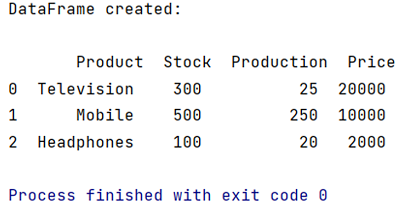# Convert List of Dictionaries to a Pandas DataFrame

In this tutorial, we will learn how to convert a list of dictionaries to a panda DataFrame with the help of example? By Pranit Sharma Last updated : April 12, 2023

## Overview

DataFrames are 2-dimensional data structures in Pandas. DataFrames consist of rows, columns, and the data. In the real world, CSV files are imported and then converted into DataFrames, but DataFrame can be created with the help of Python dictionaries, Python lists, or a list of dictionaries. Here, we are going to learn how can we convert a list of dictionaries into Pandas DataFrame?

## Pandas Convert List of Dictionaries to DataFrame

To convert a list of dictionaries to a Pandas DataFrame, use pandas.DataFrame() method and pass list of dictionaries as the parameter. This will create a DataFrame with the list of dictionaries.

### Syntax

```df = pd.DataFrame(data)
# Here, data is the list of dictionaries
```

Let us understand with the help of an example.

## Example to Convert List of Dictionaries to a Pandas DataFrame

```# Importing pandas package
import pandas as pd

# Creating a list of dictionary
data=[{'Product':'Television','Stock':300,'Production':25,'Price':20000},
{'Product':'Mobile','Stock':500,'Production':250,'Price':10000},

# Converting a list of dictionary into DataFrame
# by using pd.DataFrame method
# 'data' will be passed as a parameter inside
# DataFrame() method
df = pd.DataFrame(data)

# Display the DataFrame
print("\nDataFrame created:\n\n",df)
```

### Output

```
DataFrame created:

Price     Product  Production  Stock
0  20000  Television          25    300
1  10000      Mobile         250    500
```

### Output (Screenshot)